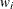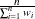### WEIGHT Statement

WEIGHT variable </ option> ;

The WEIGHT statement specifies a variable to supply weighting values to use for each observation in estimating parameters. The log likelihood for each observation is multiplied by the corresponding weight variable value.

If the weight of an observation is nonpositive, that observation is not used in the estimation.

The following option can be added to the WEIGHT statement after a slash (/).

NONORMALIZE

does not normalize the weights. By default, the weights are normalized so that they add up to the actual sample size. Weightsare normalized by multiplying them by, whereis the sample size. If the weights are required to be used as is, then specify the NONORMALIZE option.